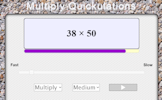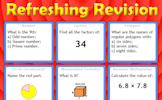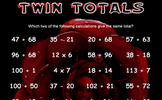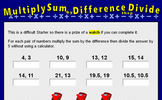# Straight line graphs

### Term 1 starting in week 1 :: Estimated time: 2 weeks

• Lines parallel to the axes, $$y = x$$ and $$y = - x$$
• Using tables of values
• Compare intercepts
• Understand and use $$y = mx + c$$
• Write an equation in the form $$y = mx + c$$
• Find the equation of a line from a graph
• Interpret gradient and intercepts of real-life graphs

For higher-attaining pupils:

• Model real-life graphs involving inverse proportion
• Explore perpendicular lines

This page should remember your ticks from one visit to the next for a period of time. It does this by using Local Storage so the information is saved only on the computer you are working on right now.

## Lesson Starters

Here are some suggestions for whole-class, projectable resources which can be used at the beginnings of each lesson in this block.

### 1st Lesson#### BTS

You have four minutes to write down as many equations as you can involving B, T and S.

### 2nd Lesson#### Red Lines

Either estimate the lengths of the red lines or, if you know how, calculate how long they are.

### 3rd Lesson#### Multiply Quickulations

Random multiplications appear on screen every few seconds.

### 4th Lesson#### Refreshing Revision

It is called Refreshing Revision because every time you refresh the page you get different revision questions.

### 5th Lesson#### Cunning Code

Decode the message by doing the calculations given for each letter.

### 6th Lesson#### Mult Sum Diff Div

For each pair of numbers multiply the sum by the difference then divide the answer by 5.

Some of the Starters above are to reinforce concepts learnt, others are to introduce new ideas while others are on unrelated topics designed for retrieval practice or and opportunity to develop problem-solving skills.

White Rose ResourcesEnd of block assessments provide a quick progress check at the end of each block of learning to make sure students have understood the content covered. This Scheme of Learning was produced by White Rose Maths and is used here with permission granted on 30th June 2021.For All: